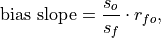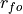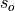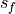# climpred.metrics._bias_slope¶

climpred.metrics._bias_slope(forecast, verif, dim=None, **metric_kwargs)[source]

Bias slope between verification data and forecast standard deviations.whereis the Pearson product-moment correlation between the forecast and the verification data andandare the standard deviations of the verification data and forecast over the experimental period, respectively.

Parameters
• forecast (xarray object) – Forecast.

• verif (xarray object) – Verification data.

• dim (str) – Dimension(s) to perform metric over.

• metric_kwargs (dict) – see pearson_r() and

:param std():

Details:
 minimum 0.0 maximum ∞ perfect 1.0 orientation negative
Reference:

Example

>>> HindcastEnsemble.verify(metric='bias_slope', comparison='e2o',
...     alignment='same_verifs', dim='init')
<xarray.Dataset>